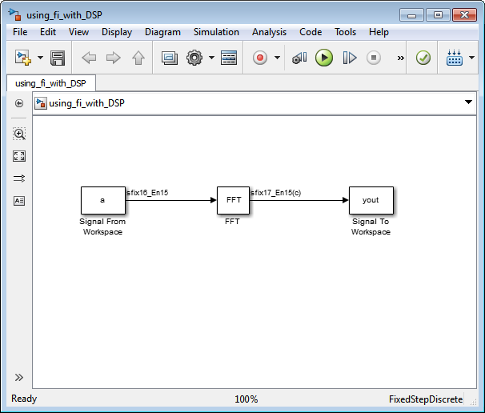## fi Objects with DSP System Toolbox

### Reading Fixed-Point Signals from the Workspace

You can read fixed-point data from the MATLAB® workspace into a Simulink® model using the Signal From Workspace and Triggered Signal From Workspace blocks from DSP System Toolbox™ software. Enter the name of the defined `fi` variable in the Signal parameter of the Signal From Workspace or Triggered Signal From Workspace block.

### Writing Fixed-Point Signals to the Workspace

Fixed-point output from a model can be written to the MATLAB workspace via the To Workspace or Triggered To Workspace block from the blockset. The fixed-point data is always written as a 2-D or 3-D array.

### Note

To write fixed-point data to the MATLAB workspace as a `fi` object, select the Log fixed-point data as a fi object check box on the Signal To Workspace or Triggered To Workspace block dialog. Otherwise, fixed-point data is converted to `double` and written to the workspace as `double`.

For example, you can use the following code to create a `fi` object in the MATLAB workspace. You can then use the Signal From Workspace block to bring the data into a Simulink model.

```a = fi([sin(0:10)' sin(10:-1:0)']) a = 0 -0.5440 0.8415 0.4121 0.9093 0.9893 0.1411 0.6570 -0.7568 -0.2794 -0.9589 -0.9589 -0.2794 -0.7568 0.6570 0.1411 0.9893 0.9093 0.4121 0.8415 -0.5440 0 DataTypeMode: Fixed-point: binary point scaling Signedness: Signed WordLength: 16 FractionLength: 15 ```

The Signal From Workspace block in the following model has these settings:

• Signal`a`

• Sample time`1`

• Samples per frame`2`

• Form output after final data value by```Setting to zero```

The following parameters in the Solver pane of the Model Configuration Parameters dialog have these settings:

• Start time`0.0`

• Stop time`10.0`

• Type`Fixed-step`

• Solver```Discrete (no continuous states)```

• Fixed step size (fundamental sample time)`1.0`

Remember, to write fixed-point data to the MATLAB workspace as a `fi` object, select the Log fixed-point data as a fi object check box on the Signal To Workspace block dialog. Otherwise, fixed-point data is converted to `double` and written to the workspace as `double`.The Signal To Workspace block writes the result of the simulation to the MATLAB workspace as a `fi` object.

```yout = (:,:,1) = 0.8415 -0.1319 -0.8415 -0.9561 (:,:,2) = 1.0504 1.6463 0.7682 0.3324 (:,:,3) = -1.7157 -1.2383 0.2021 0.6795 (:,:,4) = 0.3776 -0.6157 -0.9364 -0.8979 (:,:,5) = 1.4015 1.7508 0.5772 0.0678 (:,:,6) = -0.5440 0 -0.5440 0 DataTypeMode: Fixed-point: binary point scaling Signedness: Signed WordLength: 17 FractionLength: 15 ```
##### Support평가판 신청